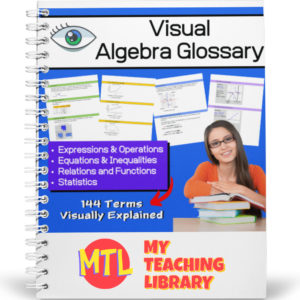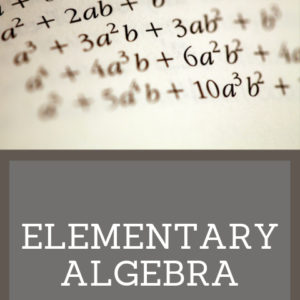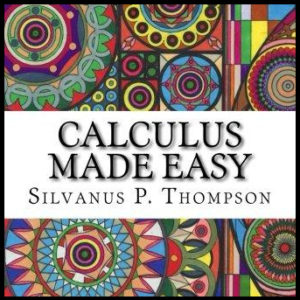Showing all 18 results

• Sale!\$7.00 \$5.50

This consumer math bundle will teach students about both simple and compound interest and give them the practice they need to help them master solving word problems!

Includes:

•\$1.50

Students will complete a crossword puzzle by answering compound interest word problems. Also included, an optional number search puzzle which will provide an answer bank to use when completing the crossword puzzle! Answer Keys provided.

Sample problem: How much interest does a \$472 investment earn at 7% compounded annually over eight years?

•\$1.50

Students will complete a crossword puzzle by answering simple interest word problems. Also included, an optional number search puzzle which will provide an answer bank to use when completing the crossword puzzle! Answer Keys provided.

Sample problem: If you put \$5.62 in a saving account that pays 3% for eight years, what is the amount of money you will have at the end of the eight years?

•\$4.00

This consumer math resource has been designed to help teach students and give them the practice they need to master the concepts taught.

The first two pages will explain interest (simple and compound) and give students the formulas they will need to solve the word problems. There are 60 word problems in all. 3 sets of 10 simple interest problems and 3 sets of 10 compound interest problems. Answer keys are provided.

•\$15.00

Give your Algebra I and Algebra II students a tool they can really use! This Visual Algebra Glossary will be an invaluable resource giving them explanations, definitions and examples of 144 terms. Easy to follow and understand, students will turn to this resource while learning new concepts, completing work and studying for exams.

There are four color coded sections, each with an term index:

• – Expressions & Operations
• – Equations & Inequalities
• – Relations & Functions
• – Statistics

73 pages

•\$10.00

Elementary Algebra is designed to meet the scope and sequence requirements of a a full year of elementary algebra. Each topic builds upon previously developed material to demonstrate the cohesiveness and structure of mathematics. Topics studied:

Arithmetic Review
Real Numbers
Algebraic Expressions & Equations
Linear Equations & Inequalities
Polynomials
Graphing Linear Equations & Inequalities
Rational Expressions
Roots, Radicals and Square Root Equations
Systems of Linear Equations

•\$3.00

Calculus Made Easy is a book on infinitesimal calculus originally published in 1910 by Silvanus P. Thompson, considered a classic and elegant introduction to the subject.

•\$3.50

Written in 1895, this text includes over 1000 exercises for practice. Topics covered include operations, factoring, fractions, complex fractions, solving equations, and solving simultaneous equations. Answers are provided at the end of the book.

•\$9.99

This algebra textbook teaches students the following:

• Pre-Algebra
• Solving Linear Equations
• Graphing
• Inequalities
• Systems of Equations
• Polynomials
• Factoring
• Rational Expressions
• Functions

•\$3.00
Here are 3 colorful “Geometry Gem” posters to display in your classroom or to place in a math, learning center as a reference.
See description below for a list of what is included!
•\$5.00

121 Math Posters – Each displaying a math term and explanation!

Designed for 4th – 12th Grades

•\$19.99

Complete High School Geometry course

•\$14.99

•\$3.00

About the book: 430 puzzles (answers provided), problems, paradoxes, and brain-teasers presented by a master of mathematical ingenuity.Virtually every sort of mathematical or logical poser is included in this extraordinary collection — problems concerning the manipulation of numbers; unicursal and route problems; moving counter puzzles; locomotion and speed problems; measuring, weighing, and packing problems; clock puzzles; combination and group problems. (See more in description below)

•\$5.00

Here are 250 word problems with answer keys. There are 25 sets, each with 10 problems each.

•\$4.00

This 123 page math resource will help students who are learning (or struggling to understand) algebra better by giving detailed explanations of related terminology and concepts. This book as been categorized as curriculum because of the detailed explanations of the topics. It however, is for instructional use only and does not include student problems.

(See description below for covered topics)

•\$9.99

A step by step Geometry curriculum to teach students with ease. Students will learn about angles, lines, triangles, polygons,quadrilaterals, prisms, coordinate geometry, slope and more!

•\$9.99

Help students prepare for the Math SAT with this resource. This 254 page workbook will help students with…

• – Techniques and Strategies
• – Numbers & Operations Review
• – Algebra Review
• – Geometry Review
• – Problem Solving
• – 3 Practice Tests – with answer key and explanations
• – Math glossary